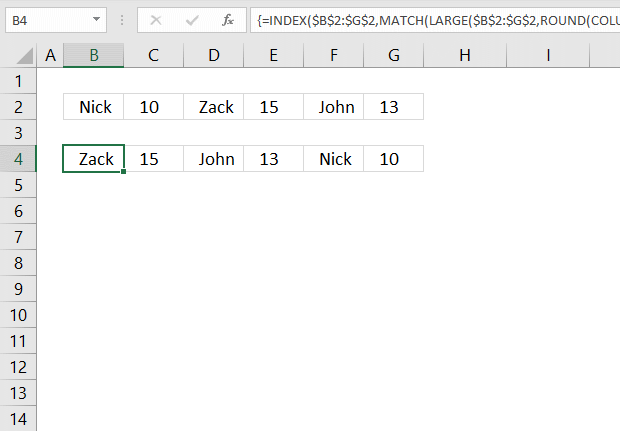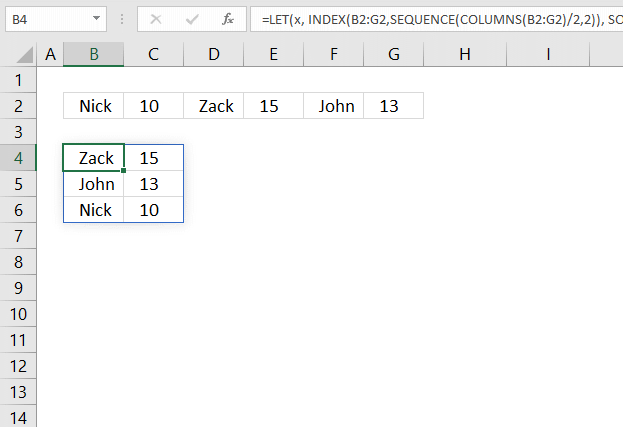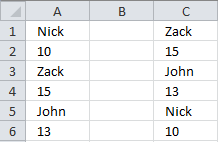Author: Oscar Cronquist Article last updated on February 08, 2023This article demonstrates a formula that sorts items arranged horizontally based on the adjacent numbers, every other column contains a number.

The image above shows the data in cell range B2:G2 and the array formula in cell B4 sorts the items based on the adjacent number from large to small.

Having data arranged like the image above shows is not something I recommend, it is far better to have the items in one column and the corresponding numbers in the next column.

You can then sort data much easier using the built-in Filter tool or convert the data to an Excel Table which gives you a lot of extra features like formatting and so on.

Denisa asks: I have a problem and I can't figure it out, even if I'm searching for 2 days.
I have the following situation:
A1, B1, C1, D1, E1, F1
where
A1 = nick
b1 = 10
c1 = zack
d1 = 15
e1 - john
f1 = 13
what formula should i use to get them ordered counting the numbers but names still being associated, like this:
a1 = zack
b1 = 15
c1 = john
d1 = 13
e1 = nick
f1 = 10## 1. Sort items by adjacent number in every other value - Array formulaArray formula in cell B4:

=INDEX(\$B\$2:\$G\$2,MATCH(LARGE(\$B\$2:\$G\$2,ROUND(COLUMN(A1)*0.5,0)),\$B\$2:\$G\$2,0)-MOD(COLUMN(A1),2))

### 1.1 How to enter an array formula

1. Type the above formula in cell B4.
2. Press and hold CTRL + SHIFT keys simultaneously.
3. Press Enter once.
4. Release all keys.

Copy cell B4 and paste to cells to the right as far as needed.

### 1.2 Explaining formula in cell B4

#### Step 1 - Calculate column number

The COLUMN function returns the column number of the top-left cell of a cell reference.

COLUMN(reference)

COLUMN(A1)*0.5

becomes

1*0.5

The asterisk character is a mathematical operator, it multiplies two numbers.

1*0.5 equals 0.5.

#### Step 2 - Round number to nearest integer

The ROUND function rounds a number based on the number of digits you specify.

ROUND(numbernum_digits)

ROUND(COLUMN(A1)*0.5,0)

becomes

ROUNDD(0.5)

and returns 1.

#### Step 3 - Extract k-th largest number

The LARGE function calculates the k-th largest value from an array of numbers.

LARGE(arrayk)

The LARGE function ignores text values and blanks.

LARGE(\$B\$2:\$G\$2, ROUND(COLUMN(A1)*0.5, 0))

becomes

LARGE(\$B\$2:\$G\$2, 1)

becomes

LARGE({"Nick", 10, "Zack", 15, "John", 13}, 1)

and returns 15. 15 is the largest number in the array.

#### Step 4 - Find relative position

The MATCH function returns the relative position of an item in an array or cell reference.

MATCH(lookup_value, lookup_array, [match_type])

MATCH(LARGE(\$B\$2:\$G\$2,ROUND(COLUMN(A1)*0.5,0)),\$B\$2:\$G\$2,0)

becomes

MATCH(15, \$B\$2:\$G\$2,0)

becomes

MATCH(15, {"Nick", 10, "Zack", 15, "John", 13},0)

and returns 4. 15 is the fourth value in the array.

#### Step 5 - Calculate a number sequence that alternates between 0 (zero) and 1.

The MOD function returns the remainder after a number is divided by a divisor.

MOD(numberdivisor)

MOD(COLUMN(A1),2)

becomes

MOD(1,2)

and returns 1.

#### Step 6 - Calculate position

MATCH(LARGE(\$B\$2:\$G\$2,ROUND(COLUMN(A1)*0.5,0)),\$B\$2:\$G\$2,0)-MOD(COLUMN(A1))

becomes

4-MOD(COLUMN(A1))

becomes

4-1

and returns 3.

#### Step 7 - Get value

The INDEX function returns a value from a cell range based on a row and column number.

INDEX(array, [row_num], [column_num])

INDEX(\$B\$2:\$G\$2,MATCH(LARGE(\$B\$2:\$G\$2,ROUND(COLUMN(A1)*0.5,0)),\$B\$2:\$G\$2,0)-MOD(COLUMN(A1),2))

becomes

INDEX(\$B\$2:\$G\$2,3)

becomes

INDEX({"Nick", 10, "Zack", 15, "John", 13},3)

and returns "Zack" in cell B4.

## 2. Sort items by adjacent number in every other value - Excel 365 formulaThe image above demonstrates a dynamic array formula in cell B4 that sorts and rearranges values based on a horizontal cell range.

Excel 365 formula in cell B4:

=LET(x, INDEX(B2:G2, SEQUENCE(COLUMNS(B2:G2)/2, 2)), SORTBY(x, INDEX(x, 0, 2), -1))

The formula above is entered as a regular formula and it works only in Excel 365.

## 3. Sort items by adjacent number in every other value verticallyThis formula returns data vertically:

=INDEX(\$A\$1:\$A\$6, MATCH(LARGE(\$A\$1:\$A\$6, ROUND(ROW(A1)*0.5, 0)), \$A\$1:\$A\$6, 0)-MOD(ROW(A1), 2))

### Get the Excel file